Conduction: One Dimensional - 2

# Conduction: One Dimensional - 2 Notes | Study Heat Transfer - Mechanical Engineering

## Document Description: Conduction: One Dimensional - 2 for Mechanical Engineering 2022 is part of Steady State Heat Conduction for Heat Transfer preparation. The notes and questions for Conduction: One Dimensional - 2 have been prepared according to the Mechanical Engineering exam syllabus. Information about Conduction: One Dimensional - 2 covers topics like and Conduction: One Dimensional - 2 Example, for Mechanical Engineering 2022 Exam. Find important definitions, questions, notes, meanings, examples, exercises and tests below for Conduction: One Dimensional - 2.

Introduction of Conduction: One Dimensional - 2 in English is available as part of our Heat Transfer for Mechanical Engineering & Conduction: One Dimensional - 2 in Hindi for Heat Transfer course. Download more important topics related with Steady State Heat Conduction, notes, lectures and mock test series for Mechanical Engineering Exam by signing up for free. Mechanical Engineering: Conduction: One Dimensional - 2 Notes | Study Heat Transfer - Mechanical Engineering
 1 Crore+ students have signed up on EduRev. Have you?

Illustration 2.1
The two sides of a wall (2 mm thick, with a cross-sectional area of 0.2 m2) are maintained at 30oC and 90oC. The thermal conductivity of the wall material is 1.28 W/(m·oC). Find out the rate of heat transfer through the wall?
Solution 2.1
Assumptions
2. Thermal conductivity is constant for the temperature range of interest
3. The heat loss through the edge side surface is insignificant
4. The layers are in perfect thermal contact
Given,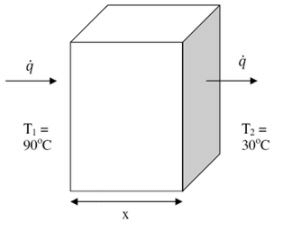Fig. 2.4: Illustration 2.1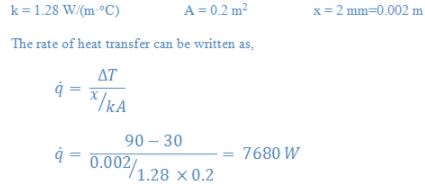Illustration 2.2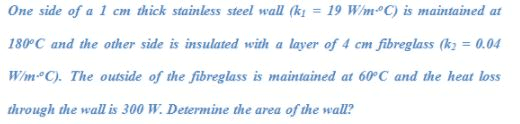Solution 2.2
Assumptions:
2. Thermal conductivity is constant for the temperature range of interest.
3. The heat loss through the edge side surface is insignificant.
4. The layers are in perfect thermal contact.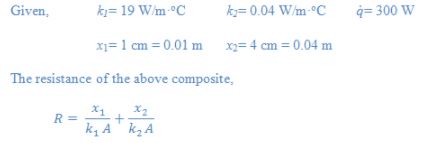On putting all the known values,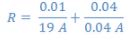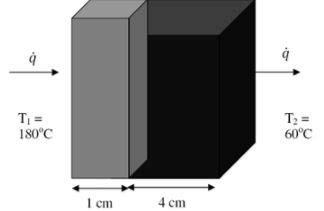Fig. 2.5: Illustration 2.2

Thus,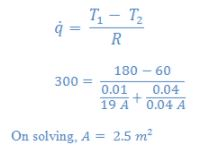The previous discussion showed the resistances of different layers. Now to understand the concept of equivalent resistance, we will consider the geometry of a composite as shown in fig.2.6a.

The wall is composed of seven different layers indicated by 1 to 7. The interface temperatures of the composite are T1 to T5 as shown in the fig.2.6a. The equivalent electrical circuit of the above composite is shown in the fig 2.6b below,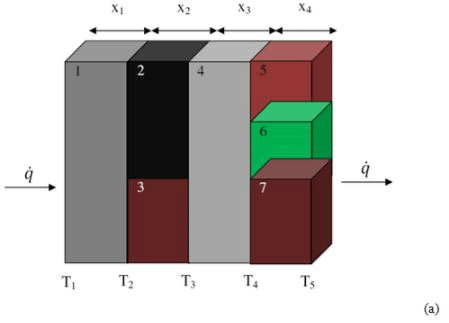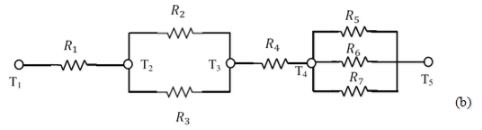Fig.2.6. (a) Composite wall, and (b) equivalent electrical circuit

The equivalent resistance of the wall will be,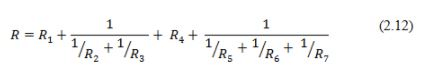where,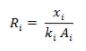Therefore, at steady state the rate of heat transfer through the composite can be represented by,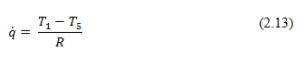where, R is the equivalent resistance.

The document Conduction: One Dimensional - 2 Notes | Study Heat Transfer - Mechanical Engineering is a part of the Mechanical Engineering Course Heat Transfer.
All you need of Mechanical Engineering at this link: Mechanical Engineering

## Heat Transfer

58 videos|70 docs|85 tests
 Use Code STAYHOME200 and get INR 200 additional OFF

## Heat Transfer

58 videos|70 docs|85 tests

Track your progress, build streaks, highlight & save important lessons and more!

,

,

,

,

,

,

,

,

,

,

,

,

,

,

,

,

,

,

,

,

,

;